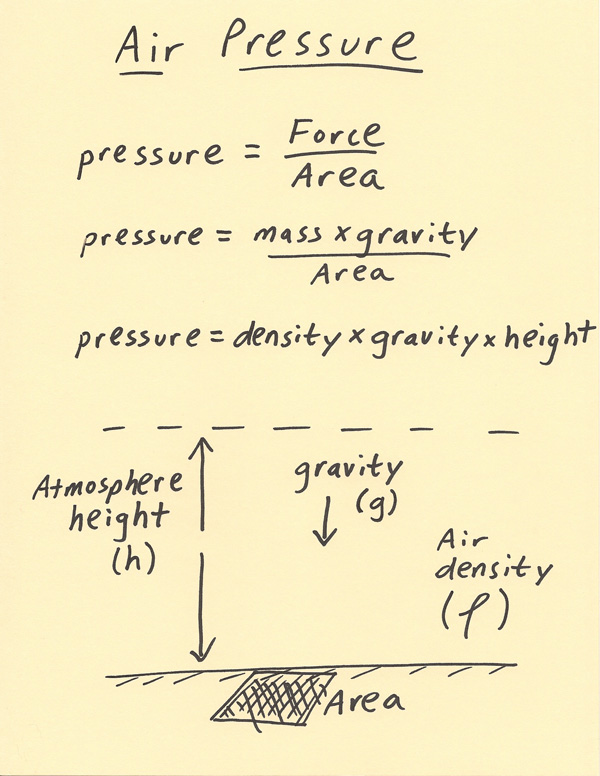[--MAIN HOME--] [--ALL HABYHINTS--] [--FACEBOOK PAGE--]

 HABYTIME MINI LECTURE 59: SURFACE AIR PRESSURE

METEOROLOGIST JEFF HABY

Air pressure is the force divided by area. Force is mass times gravity. Another way of looking at this is that pressure is gravity’s influence on the mass of the air above an area. Since mass over area is equivalent to density times height, another way of looking at air pressure is that pressure is the air density times gravity times height. Each of these equations is drawn out below. Regardless of the equation used, the units of pressure in the SI system work out to Newtons (kgms^-2) divided by area, for final units of kgm^-1s^-2. This derived unit is called a Pascal.

The diagram below shows a visual of how the concepts relate to each other to produce air pressure. The height of the atmosphere (h) is shown on the diagram. In reality, there is no top to the atmosphere but rather it merges into outer space. To compensate for this, to get a more accurate sense of the pressure, the atmosphere is divided into layers with height. Each layer going higher up contributes less and less to the total pressure since pressure decreases rapidly with height. At very high altitudes, the contribution to the atmospheric surface air pressure is near 0 since there is very little air very high aloft. For our purposes, we can label the top of the atmosphere at a very high altitude aloft. Gravity is forcing this air toward the ground. This downward gravitational force is basically a constant. Gravity does not push the air all the way to the ground surface since the upward directed vertical pressure gradient force has come into balance with gravity. The final factor is the density of the air. Since the density decreases rapidly with height, this is another reason to divide the atmosphere into layers and find the average density of each layer. To integrate the air pressure, progressively thinner and thinner slices of the atmosphere can be analyzed for their density contribution to calculate the total air pressure. The derived unit of area used in the SI system is the meter squared. Thus, the air density above a meter square of area under the influence of Earth’s gravity is what produces the surface air pressure. The primary factor that will cause the air pressure to vary at the surface is changing the air density. Air density varies with changes in temperature and humidity. It also changes due to convergence and divergence which causes an uplifting or sinking of air depending on where it takes place in the atmosphere. Here are various factors and the usual impact they have on air pressure, assuming all other factors are held constant. For example, if temperature warms it is assumed below there are no other factors such as rising air that is causing the pressure to change. In reality this is often not the case but the information below is constructive in understanding the isolated individual contributions that cause the surface air pressure to vary:

Warm the air: decreases surface air pressure (since warmer air is less dense)
Cool the air: increases surface air pressure (since cooler air is denser)
Rising air: decreases surface air pressure (since air mass is being removed)
Sinking air: increases surface air pressure (since air mass is being added)
Add moisture to the air: decreases air pressure (since humid air is less dense)
Dry the air: increases air pressure (since dry air is denser)